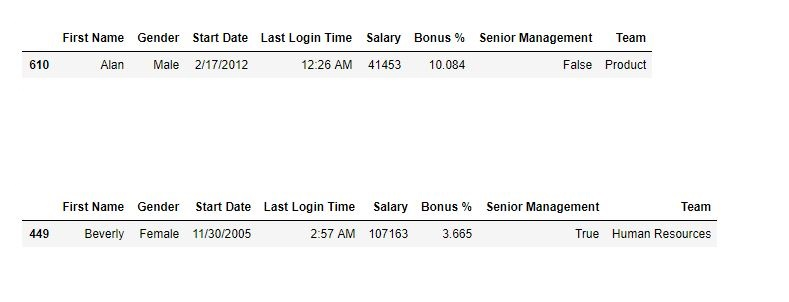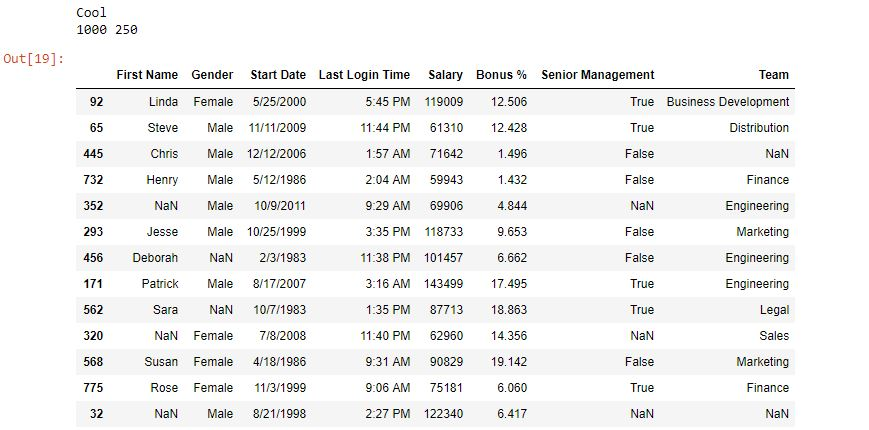Related Articles

# Python | Pandas Dataframe.sample()

• Difficulty Level : Easy
• Last Updated : 24 Apr, 2020

Python is a great language for doing data analysis, primarily because of the fantastic ecosystem of data-centric python packages. Pandas is one of those packages and makes importing and analyzing data much easier.

Pandas `sample()` is used to generate a sample random row or column from the function caller data frame.

Syntax:

DataFrame.sample(n=None, frac=None, replace=False, weights=None, random_state=None, axis=None)

Parameters:

n: int value, Number of random rows to generate.
frac: Float value, Returns (float value * length of data frame values ). frac cannot be used with n.
replace: Boolean value, return sample with replacement if True.
random_state: int value or numpy.random.RandomState, optional. if set to a particular integer, will return same rows as sample in every iteration.
axis: 0 or ‘row’ for Rows and 1 or ‘column’ for Columns.

Return type: New object of same type as caller.

Example #1: Random row from Data frame

In this example, two random rows are generated by the .sample() method and compared later.

 `# importing pandas package``import` `pandas as pd`` ` `# making data frame from csv file ``data ``=` `pd.read_csv(``"employees.csv"``)`` ` `# generating one row ``row1 ``=` `data.sample(n ``=` `1``)`` ` `# display``row1`` ` `# generating another row``row2 ``=` `data.sample(n ``=` `1``)`` ` `# display``row2`

Output:
As shown in the output image, the two random sample rows generated are different from each other.Example #2: Generating 25% sample of data frame
In this example, 25% random sample data is generated out of the Data frame.

 `# importing pandas package``import` `pandas as pd`` ` `# making data frame from csv file ``data ``=` `pd.read_csv(``"employees.csv"``)`` ` `# generating one row ``rows ``=` `data.sample(frac ``=``.``25``)`` ` `# checking if sample is 0.25 times data or not`` ` `if` `(``0.25``*``(``len``(data))``=``=` `len``(rows)):``    ``print``( ``"Cool"``)``    ``print``(``len``(data), ``len``(rows))`` ` `# display``rows`

Output:
As shown in the output image, the length of sample generated is 25% of data frame. Also the sample is generated randomly.Attention geek! Strengthen your foundations with the Python Programming Foundation Course and learn the basics.

To begin with, your interview preparations Enhance your Data Structures concepts with the Python DS Course. And to begin with your Machine Learning Journey, join the Machine Learning – Basic Level Course

My Personal Notes arrow_drop_up